International
Tables for
Crystallography
Volume B
Reciprocal space
Edited by U. Shmueli

International Tables for Crystallography (2010). Vol. B, ch. 3.2, pp. 416-417   | 1 | 2 |
https://doi.org/10.1107/97809553602060000769

Appendix A3.2.1.

R. E. Marsha* and V. Schomakerb

aThe Beckman Institute–139–74, California Institute of Technology, 1201 East California Blvd, Pasadena, California 91125, USA, and  bDepartment of Chemistry, University of Washington, Seattle, Washington 98195, USA
Correspondence e-mail:  rem@xray.caltech.edu

Consider n atoms at observed vector positions(expressed in Cartesians), n constraints (each adjusted position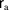– a' for adjusted' – must be on the plane) andadjustable parameters (3ncomponents and the 3 components of the vectorof reciprocal intercepts of the plane), so that the problem hasdegrees of freedom. The weight matricesmay be differently anisotropic for each atom, but there are no interatomic correlations. As before, square brackets,', represent the Gaussian sum over all atoms, usually suppressing the atom indices. We also write, not theof Section 3.2.2, for the Lagrange multipliers (one for each atom);for the direction cosines of the plane normal; and d for the perpendicular origin-to-plane distance.

As before,is the reciprocal of the atomic error matrix:(correspondingly,but' is no longer the' of Section 3.2.2.The appropriate least-squares sum is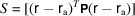and the augmented sum for applying the method of Lagrange multipliers is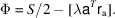is to be minimized with respect to variations of the adjusted atom positionsand plane reciprocal intercepts, leading to the equations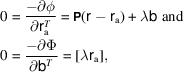subject to the plane conditions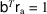, each atom, with,. These equations are nonlinear.

A convenient solution runs as follows: first multiply the first equation byand solve for the adjusted atom positions in terms of the Lagrange multipliersand the reciprocal interceptsof the plane; then multiply that result byapplying the plane conditions, and solve for the's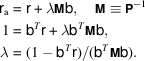Next insert these values for the's and's into the second equation: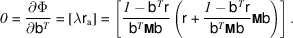This last equation,, is to be solved for. It is highly nonlinear:. One can proceed to a first approximation by writing; i.e.,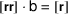, in dyadic notation., all atoms;in the multiplier ofA linear equation in, this approximation usually works well.6 We have also used the iterative Frazer, Duncan & Collar eigenvalue solution as described by SWMB (1959), which works even when the plane passes exactly through the origin. To continue the solution of the nonlinear equations, by linearizing and iterating, writein the formsolve for, resetto, etc., until the desired degree of convergence oftoward zero has been attained. Byis meant the partial derivative of the above expression forwith respect to, as detailed in the next paragraph.

In the Fortran program DDLELSP (double precision Deming Lagrange, with error estimates, least-squares plane, written to explore this solution) the preceding equation is recast aswithThe usual goodness of fit, GOF2 in DDLELSP, evaluates to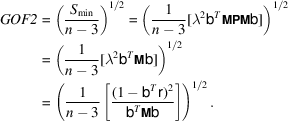This is only an approximation, because the residualsare not the differences between the observations and appropriate linear functions of the parameters, nor are their variances (the's) independent of the parameters (or, in turn, the errors in the observations).

We ask also about the perpendicular distances, e, of atoms to plane and the mean-square deviationto be expected in e.Hereandare the errors inand. Neglecting' then leads toWe have, butandperhaps still need to be evaluated.

References

Schomaker, V., Waser, J., Marsh, R. E. & Bergman, G. (1959). To fit a plane or a line to a set of points by least squares. Acta Cryst. 12, 600–604.